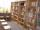# Passenger boat

Two-fifths of the passengers in the passenger boat were boys. 1/3 of them were girls and the rest were adult. If there were 60 passengers in the boat, how many more boys than adult were there?

Result

x =  8

#### Solution:Leave us a comment of example and its solution (i.e. if it is still somewhat unclear...):Be the first to comment!#### To solve this verbal math problem are needed these knowledge from mathematics:

Need help calculate sum, simplify or multiply fractions? Try our fraction calculator.

## Next similar examples:

1. TeacherTeacher Rem bought 360 pieces of cupcakes for the outreach program of their school. 5/9 of the cupcakes were chocolate flavor and 1/4 wete pandan flavor and the rest were vanilla flavor. How much more chocolate flavor cupcakes than vanilla flavor?
2. School libraryThe school library contains 6300 books. With this constitutes 7% of professional books for teachers 18% interest books and encyclopedias for pupils and the rest of the fairy tales. How much are which books?
3. Grandmother and grandfatherGrandmother baked cakes. Grandfather ate half, then quarter of the rest ate Peter and Paul ate half of rest. For parents left 6 cakes. How many cakes maked the grandmother?
4. ZdeněkZdeněk picked up 15 l of water from a 100-liter full-water barrel. Write a fraction of what part of Zdeněk's water he picked.
5. Pizza 4Marcus ate half pizza on monday night. He than ate one third of the remaining pizza on Tuesday. Which of the following expressions show how much pizza marcus ate in total?
6. Lengths of the poolMiguel swam 6 lengths of the pool. Mat swam 3 times as far as Miguel. Lionel swam 1/3 as far as Miguel. How many lengths did mat swim?
7. In fractionsAn ant climbs 2/5 of the pole on the first hour and climbs 1/4 of the pole on the next hour. What part of the pole does the ant climb in two hours?
8. Cupcakes 2Susi has 25 cupcakes. She gives 4/5. How much does she have left?
9. Write 2Write 791 thousandths as fraction in expanded form.
10. WithdrawalIf I withdrew 2/5 of my total savings and spent 7/10 of that amount. What fraction do I have in left in my savings?
11. Fraction to decimalWrite the fraction 3/22 as a decimal.
12. Mixed2improperWrite the mixed number as an improper fraction. 166 2/3
13. Fraction and a decimalWrite as a fraction and a decimal. One and two plus three and five hundredths
14. NumberI think the number. If I add to its third seven I get same as when to its quarter add 8. Which is the number?
15. Equation 15Solve equation with variables on both sides:
16. Unknown number 6Determine x if 1/6 of x is equal to 2/5 of the number 24.
17. Sum of fractionsWhat is the sum of 2/3+3/5?